## Example 26.24 Fitting a Latent Growth Curve Model

Latent factors in structural equation modeling are constructed to represent important unobserved hypothetical constructs. However, with some manipulations latent factors can also represent random effects in models. In this example, a simple latent growth curve model is considered. You use latent factors to represent the random intercepts and slopes in the latent growth curve model.

Sixteen individuals were invited to a training program that was designed to boost self-confidence. During the training, the individuals’ confidence levels were measured at five time points: initially and four more times separated by equal intervals. The data are stored in the following SAS data set:

```data growth;
input y1 y2 y3 y4 y5;
datalines;
17.6  21.4  25.6  32.1  37.7
13.2  14.3  18.9  20.3  25.4
11.6  13.5  17.4  22.1  39.6
10.7  11.1  13.2  18.2  21.4
18.7  23.7  28.6  31.5  34.0
18.3  19.2  20.5  23.2  25.9
9.2  13.5  17.8  19.2  21.1
18.3  23.5  27.9  30.2  34.6
11.2  15.6  20.8  22.7  30.4
17.0  22.9  26.9  31.9  35.6
10.4  13.6  18.0  25.6  29.3
17.7  19.0  22.5  28.5  30.7
14.5  19.4  21.1  28.8  31.5
20.0  21.4  28.9  30.2  35.6
14.6  19.3  21.7  28.5  32.0
11.7  15.2  19.1  23.7  28.7
;
```

First, consider a simple linear regression model for the confidence levels at timedue to training. That is,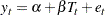where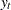represents the confidence level at time(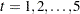),
represents the intercept,represents the slope or the effect of training,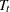represents the fixed time point at(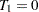and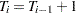), and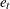is the error term at time.

This simple linear regression assumes that the effect of training (slope) and the intercept are constants for the individuals. However, individual differences are rules rather than exceptions. It is thus more reasonable to argue that an indexfor individuals should be added to the intercept and slope in the model. As a result, the following individualized regression model is derived: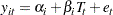where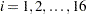. In this model, individuals are assumed to have different intercepts and slopes (regression coefficients). Note that theoreticallycould also be "individualized" as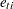in the model. But this is not done because such a model would be unnecessarily complicated without gaining additional insights in return.

Unfortunately, this individualized model with individual intercepts and slopes cannot be estimated directly. If you treat each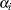and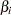as fixed parameters, you are going to have too many parameters for the model to be identified or estimable. A workable solution is to treatandin the original linear regression model as random variables instead. That is, the latent growth curve model of interest is as follows:where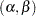is bivariate normal with unknown means, variances, and covariance. Therefore, instead of having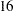intercepts andslopes to estimate in the individualized regression model, the final latent growth curve model has to estimate only two means, two variances and one covariance in the bivariate distribution of.

To use PROC CALIS to fit this latent growth curve model, the random intercept and effect are treated as if they were covarying latent factors. To make them stand out more as latent variables, the random intercept and slope are renamed as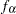and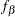in the following structural equation: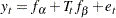whereandare bivariate-normal latent variables. This model assumes that the error distribution is time dependent (with the index). A simpler version is to make this error term invariant over time, which is then represented by the following model with constrained error variances: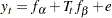This constrained model is considered first. The LINEQS modeling language is used to specify this constrained model, as shown in the following statements.

```proc calis method=ml data=growth nostand noparmname;
lineqs
y1 = 0. * Intercept + f_alpha                + e1,
y2 = 0. * Intercept + f_alpha  +  1 * f_beta + e2,
y3 = 0. * Intercept + f_alpha  +  2 * f_beta + e3,
y4 = 0. * Intercept + f_alpha  +  3 * f_beta + e4,
y5 = 0. * Intercept + f_alpha  +  4 * f_beta + e5;
variance
f_alpha f_beta,
e1-e5 = 5 * evar;
mean
f_alpha f_beta;
cov
f_alpha f_beta;
fitindex on(only)=[chisq df probchi];
run;
```

In the LINEQS model specification, f_alpha and f_beta are treated as latent factors representing the random intercept and random slope, respectively. The f_ prefix for latent factors is required as a convention in the LINEQS modeling language. See the sections Naming Variables in the LINEQS Model and Naming Variables and Parameters for details.

Notice that you need to set the ordinary (non-random) intercepts for endogenous variables to zero by the 0.*Intercept specification because non-random intercepts for observed endogenous variables are default parameters in the LINEQS model. Because you have already used f_alpha as the random intercept, you must turn off the default non-random intercept term for the observed endogenous variables y1y5. Otherwise, your latent growth curve model might be over-parameterized.

At,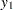represents the initial confidence measurement so that it is not subject to the random effect f_beta. The next four measurements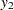,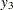,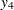, and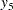are measured at time points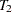,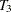,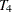, and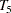, respectively. These are fixed time points with constant values,,, and, respectively, in the equations of the LINEQS statement.

The means, variances and covariances of f_alpha and f_beta are parameters in the model. The variances of these two latent variables are specified in the VARIANCE statement, while their covariance is specified in the COV statement. The means of f_alpha and f_beta are specified in the MEAN statement. Unlike the specification for the variances of e1e5. All these parameters for the latent factors are unnamed because you do not need to constrain them by references.

The error variances for e1e5 are also specified in the VARIANCE statement. Using the shorthand notation 5 * evar, the parameter name evar is repeated five times for the five error variances. This constrains the error variances for e1e5 to be equal.

You also use some special printing options in this example. In the PROC CALIS statement, the NOSTAND option is specified because standardized solution is not of interest. The reason is that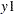-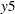were already measured on comparable scales, making standardization unnecessary for interpretations. Another printing option specified is the NOPARMNAME option in the PROC CALIS statement. This option suppresses the printing of parameter names in the output for estimation. This makes the output look more concise when you do not need to make references to the parameter names. Still another printing option used is the ON(ONLY)= option of the FITINDEX statement. This option trims down the display of fit indices to include only those listed in the option. See the FITINDEX statement for details.

Output 26.24.1 shows the fit summary table.

Output 26.24.1 Random Intercepts and Effects with Constrained Error Variances: Model Fit
Fit Summary
Chi-Square 31.4310
Chi-Square DF 14
Pr > Chi-Square 0.0048

In Output 26.24.1, the chi-square value in the fit summary table is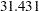(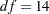,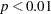), which is a statistically significant result that might indicate a poor model fit. Despite that, it is illustrative to continue to look at the main estimation results, which are shown in the following table.

Output 26.24.2 Estimation of Random Intercepts and Effects with Constrained Error Variances
Estimates for Variances of Exogenous Variables
Variable
Type
Variable Estimate Standard
Error
t Value
Latent f_alpha 13.89140 5.81540 2.38873
f_beta 0.80742 0.42198 1.91342
Error e1 3.32185 0.70031 4.74342
e2 3.32185 0.70031 4.74342
e3 3.32185 0.70031 4.74342
e4 3.32185 0.70031 4.74342
e5 3.32185 0.70031 4.74342

Covariances Among Exogenous Variables
Var1 Var2 Estimate Standard
Error
t Value
f_alpha f_beta -0.35281 1.13815 -0.30998

Mean Parameters
Variable
Type
Variable Estimate Standard
Error
t Value
Latent f_alpha 14.15875 1.02906 13.75890
f_beta 4.04813 0.27563 14.68665

In Output 26.24.2, the estimated variance of the random intercept, which is represented by the variance estimate of the latent factor f_alpha, is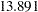(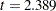). In the next row of the same table, the variance estimate of the random effect, which is represented by the variance estimate of the latent factor f_beta, is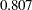(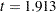).

The covariance of the random intercept and the random effect is shown in the next table for "Covariances Among Exogenous Variables." A negative estimate of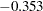is shown. This means that the initial self-confidence level and the boosting effect of training are negatively correlated. The higher the initial self-confidence level, the smaller the training effect.

In the last table for the "Mean Parameters," the estimated mean of the random intercept is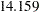, which is an estimate of the averaged initial self-confidence level. The estimated mean of random effect is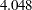, which is an estimate of the averaged training effect. They are both significantly different from zero.

Given that the model does not fit that well, perhaps you should not take the interpretations of these estimates so seriously. Knowing that the distribution of the errors might have been time-dependent, you now try to improve the fit of the model by relaxing the constraint about common error variances. You can use the following specifications:

```proc calis method=ml data=growth nostand noparmname;
lineqs
y1 = 0. * Intercept + f_alpha                + e1,
y2 = 0. * Intercept + f_alpha  +  1 * f_beta + e2,
y3 = 0. * Intercept + f_alpha  +  2 * f_beta + e3,
y4 = 0. * Intercept + f_alpha  +  3 * f_beta + e4,
y5 = 0. * Intercept + f_alpha  +  4 * f_beta + e5;
variance
f_alpha f_beta,
e1-e5;
mean
f_alpha f_beta;
cov
f_alpha f_beta;
fitindex on(only)=[chisq df probchi];
run;
```

In this new specification, there is only one change in the VARIANCE statement from the previous specification. That is, you now specify only the error variables without putting parameter names for them. This makes the variances of e1e5 free (unconstrained) parameters in the model.

Output 26.24.3 shows the model fit summary.

Output 26.24.3 Random Intercepts and Effects with Unconstrained Error Variances: Model Fit
Fit Summary
Chi-Square 11.6250
Chi-Square DF 10
Pr > Chi-Square 0.3109

The chi-square for the unconstrained model is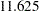(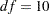,
). This indicates an acceptable model fit. The chi-square difference test can also be conducted for testing the previous constrained model against this new model. The chi-square difference is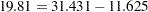. With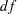=4, this chi-square difference value is statistically significant at=0.01, indicating a significant improvement of model fit by using the unconstrained model.

Output 26.24.4 shows the estimation results.

Output 26.24.4 Estimation of Random Intercepts and Effects with Unconstrained Error Variances
Estimates for Variances of Exogenous Variables
Variable
Type
Variable Estimate Standard
Error
t Value
Latent f_alpha 14.70071 5.66943 2.59298
f_beta 0.45059 0.29867 1.50867
Error e1 2.81712 1.35332 2.08164
e2 0.32213 0.46118 0.69848
e3 1.94429 0.86824 2.23935
e4 1.88569 1.21306 1.55448
e5 14.65193 5.99354 2.44462

Covariances Among Exogenous Variables
Var1 Var2 Estimate Standard
Error
t Value
f_alpha f_beta 0.35291 0.90366 0.39054

Mean Parameters
Variable
Type
Variable Estimate Standard
Error
t Value
Latent f_alpha 14.03046 1.01534 13.81851
f_beta 3.96793 0.22612 17.54781

The estimation results for the unconstrained model present a slightly different picture than the constrained model. While the estimates for the means and variances of the random intercept and the random training effect look similar in both models, estimates of the covariance between the random intercept and the random training effect are quite different in the two models. The covariance estimate is negative () in the constrained model, but it is positive (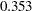) in the unconstrained model. However, because the covariance estimates are not statistically significant in both models (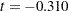and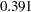, respectively), you wonder whether the current data are showing strong evidence that supports one way or another. To get a clearer picture, perhaps you need to collect more data and fit the models again to examine the significance of the covariance between the random intercept and slope.